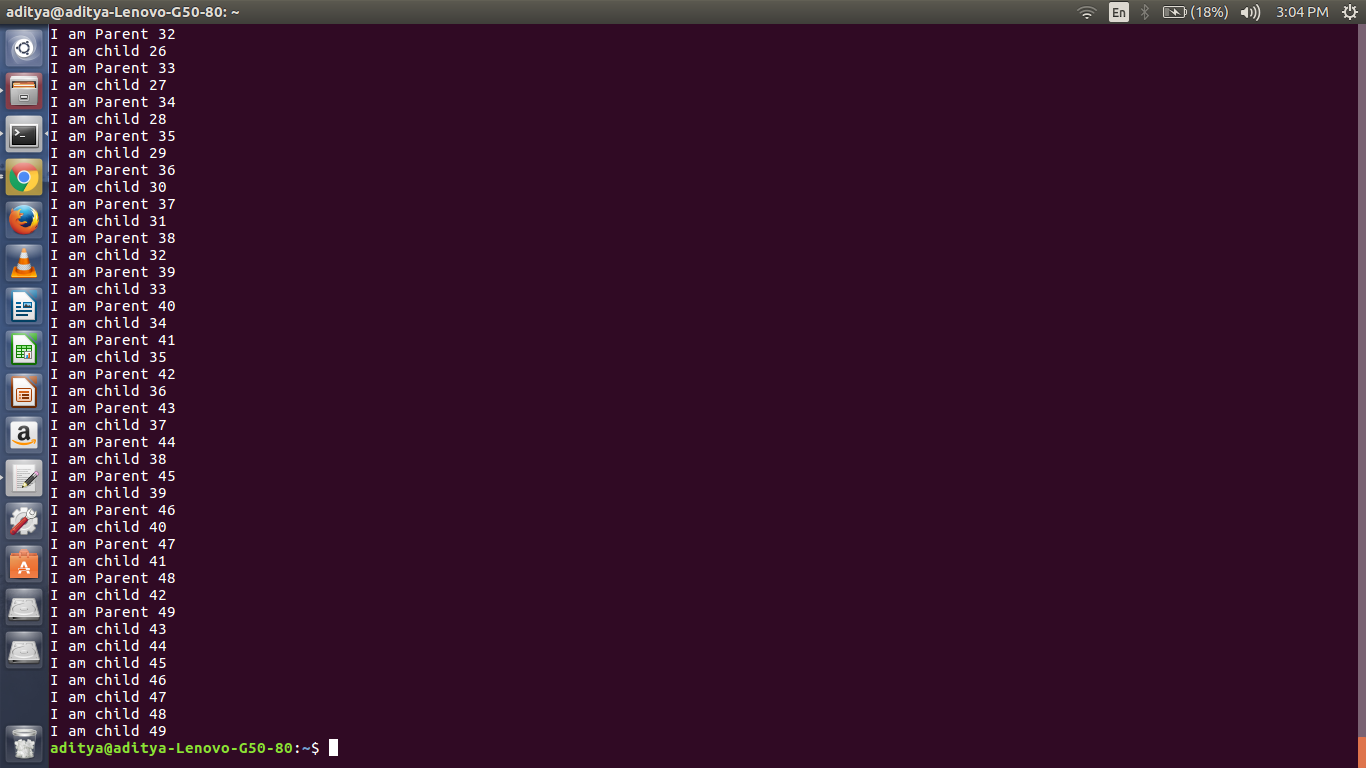# Program to show that Linux provides time sharing environment to processes

Time-sharing means sharing of computing resources among many users (processes) by means of multiprogramming and multitasking. By allowing a large number of users to interact concurrently, time-sharing dramatically lowered the cost of providing computing capability.

Many operating system including Windows, Linux and many others provides time-sharing mechanism to different processes.
Here, our task is to show that Linux provides time-sharing mechanism using a simple program.

Approach : Here, two process (parent and child) is created using fork() system call having some print statement in loop. In output we will see that print statement of these two process will execute alternatively showing time-sharing mechanism between two processes.

## Recommended: Please try your approach on {IDE} first, before moving on to the solution.

 `// C program to demonstrate that Linux is ` `// time-sharing ` `#include ` `#include ` ` `  `// Child process ` `void` `child() ` `{ ` `    ``int` `i; ` `    ``for` `(i = 0; i < 50; i++) ` `        ``printf``(``"I am child %d\n"``, i); ` `} ` ` `  `// Parent process ` `void` `parent() ` `{ ` `    ``int` `i; ` `    ``for` `(i = 0; i < 50; i++) ` `        ``printf``(``"I am Parent %d\n"``, i); ` `} ` ` `  `// Driver code ` `int` `main() ` `{ ` `    ``pid_t pid = fork(); ` ` `  `    ``// fork() error ` `    ``if` `(pid < 0) ` `        ``printf``(``"Fork Failed"``); ` ` `  `    ``// child ` `    ``else` `if` `(pid == 0) ` `        ``child(); ` ` `  `    ``// parent ` `    ``else` `        ``parent(); ` ` `  `    ``return` `0; ` `} `

Output:
In the below screenshot, we can see that both print statement is executing concurrently and not one after completion of other.